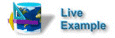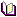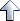Application Techniques

# Graphing Data Using a Servlet

How to build a page that loads an image that is generated by a servlet.

Details

Category

DescriptionYou can run this technique code from:

NOTE   First make sure that database is running on your localhost SilverStream ServerRelated reading

See the chapter on programming pages in the Programmer's Guide

This technique uses a servlet called srvletGraphMe to load a graph into a SilverStream page. The servlet contains all of the logic required to construct the graph image. To construct the image, the servlet uses several of the classes in the AWT. Once the image has been created, the servlet invokes the services of a class called GifEncoder, which is provided by Acme, to generate the GIF from the image.

# Loading the image from the servletThe pageRequestBegin event and the pageActionPerformed event for the Graph button both call the paintTheGraph() method, which sets the source of the image to the URL for the servlet (GraphMe). The paintTheGraph() method gets five values entered by the user on the page and then passes these values to the servlet. For the sake of brevity, much of the code in this method is not shown here:

```  ...
...
...
imgGraphMe.setSrc(getDatabaseURL().toString() +
"/GraphMe?ONE=" + m_field1 +
"&TWO=" + m_field2 +
"&THREE=" + m_field3 +
"&FOUR=" + m_field4 +
"&FIVE=" + m_field5);
```

# Importing the Acme classes into the servletThe srvletGraphMe servlet imports classes in the Acme.JPM.Encoders package, which was uploaded to the server in the Acme.jar file. This package contains the GifEncoder class:

```  ...
...
import Acme.JPM.Encoders.*;
...
```

# Coding the service method on the servletThe service method in the srvletGraphMe servlet calls a method called createGraphImage(), which creates a bar graph. Then it passes the image returned by createGraphImage() to the constructor for the GifEncoder class and calls the encode() method on the GifEncoder class:

```  synchronized(this){
AgoHttpRequestEvent request = (AgoHttpRequestEvent) req;

Image m_image = createGraphImage (request);

OutputStream out = response.getOutputStream();
GifEncoder gif = new GifEncoder (m_image, out);
gif.encode();

String mimetype = "image/gif";
response.setContentType(mimetype);
response.setStatus(response.SC_OK);
}
```

# Creating the graphThe createGraphImage() method constructs the graph image. It gets the five parameters passed to it by the SilverStream page and uses these to draw the bar graph. The createGraphImage() method uses the services of two other methods defined on the servlet, drawPanel() and drawBar():

```  // initialize the data
int iData[] = new int;
iData = new
Integer(request.getParameter("ONE")).intValue();
iData = new
Integer(request.getParameter("TWO")).intValue();
iData = new
Integer(request.getParameter("THREE")).intValue();
iData = new
Integer(request.getParameter("FOUR")).intValue();
iData = new
Integer(request.getParameter("FIVE")).intValue();

//Check for extreme data parms
for (int x=0; x<5; x++){
if (iData[x] < 0) iData[x] = 0;
if (iData[x] > 400) iData[x] = 400;
}

int iWidth = 400; int iHeight = 400;
int iXInc = 60;
double scale = (double)(iHeight-10)/(double)iHeight;

m_image = m_canvasObject.createImage(iWidth+1, iHeight+1);
m_grphGraph = m_image.getGraphics();

drawPanel (iHeight, iWidth, 10, m_grphGraph);
drawBar (20,  (int) (iData * scale),
iHeight, iWidth, 10, m_grphGraph, Color.red);
drawBar (80,  (int) (iData * scale),
iHeight, iWidth, 10, m_grphGraph, Color.cyan);
drawBar (140, (int) (iData * scale),
iHeight, iWidth, 10, m_grphGraph, Color.green);
drawBar (200, (int) (iData * scale),
iHeight, iWidth, 10, m_grphGraph, Color.magenta);
drawBar (260, (int) (iData * scale),
iHeight, iWidth, 10, m_grphGraph, Color.gray);

return m_image;

```